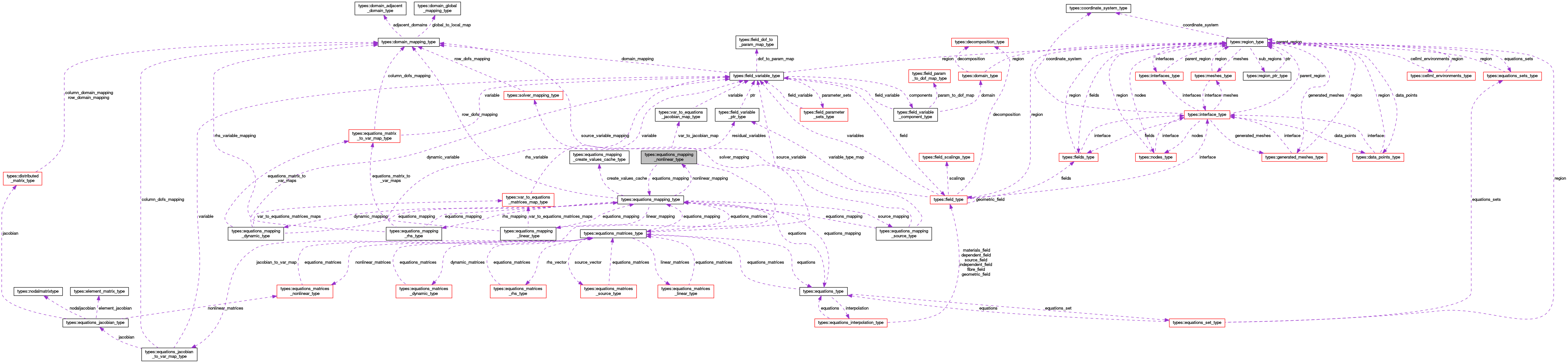OpenCMISS-Iron Internal API Documentation
types::equations_mapping_nonlinear_type Type Reference

Contains information on the equations mapping for nonlinear matrices i.e., how a field variable is mapped to residual vectors, and how the field variables are mapped to the rows and columns of the associated Jacobian matrices of the equations set of this equations mapping. There may be multiple residual variables with a Jacobian matrix for each variable. More...

Collaboration diagram for types::equations_mapping_nonlinear_type:[legend]

## Public Attributes

type(equations_mapping_type), pointer equations_mapping
A pointer to the equations mapping. More...

integer(intg) number_of_residual_variables
The number of residual variables in this mapping. More...

type(field_variable_ptr_type), dimension(:), allocatable residual_variables
RESIDUAL_VARIABLES(variable_idx). The variable_idx'th residual variable. More...

type(var_to_equations_jacobian_map_type), dimension(:), allocatable var_to_jacobian_map
VAR_TO_JACOBIAN_MAP(variable_idx). The mapping from the residual variable to the Jacobain matrix for the variable_idx'th residual variable. More...

type(equations_jacobian_to_var_map_type), dimension(:), allocatable jacobian_to_var_map
JACOBIAN_TO_VAR_MAP(jacobian_idx). The mapping from the Jacobian matrix to the residual variables for the jacobian_idx'th Jacobian. More...

real(dp) residual_coefficient
The multiplicative coefficient applied to the residual vector. More...

integer(intg), dimension(:), allocatable equations_row_to_residual_dof_map
EQUATIONS_ROW_TO_RESIDUAL_DOF_MAP(row_idx). The mapping from the row_idx'th row of the equations to the source dof. More...

## Detailed Description

Contains information on the equations mapping for nonlinear matrices i.e., how a field variable is mapped to residual vectors, and how the field variables are mapped to the rows and columns of the associated Jacobian matrices of the equations set of this equations mapping. There may be multiple residual variables with a Jacobian matrix for each variable.

Definition at line 1623 of file types.f90.

## Member Data Documentation

 type(equations_mapping_type), pointer types::equations_mapping_nonlinear_type::equations_mapping

A pointer to the equations mapping.

Definition at line 1624 of file types.f90.

 integer(intg), dimension(:), allocatable types::equations_mapping_nonlinear_type::equations_row_to_residual_dof_map

EQUATIONS_ROW_TO_RESIDUAL_DOF_MAP(row_idx). The mapping from the row_idx'th row of the equations to the source dof.

Definition at line 1630 of file types.f90.

 type(equations_jacobian_to_var_map_type), dimension(:), allocatable types::equations_mapping_nonlinear_type::jacobian_to_var_map

JACOBIAN_TO_VAR_MAP(jacobian_idx). The mapping from the Jacobian matrix to the residual variables for the jacobian_idx'th Jacobian.

Definition at line 1628 of file types.f90.

 integer(intg) types::equations_mapping_nonlinear_type::number_of_residual_variables

The number of residual variables in this mapping.

Definition at line 1625 of file types.f90.

 real(dp) types::equations_mapping_nonlinear_type::residual_coefficient

The multiplicative coefficient applied to the residual vector.

Definition at line 1629 of file types.f90.

 type(field_variable_ptr_type), dimension(:), allocatable types::equations_mapping_nonlinear_type::residual_variables

RESIDUAL_VARIABLES(variable_idx). The variable_idx'th residual variable.

Definition at line 1626 of file types.f90.

 type(var_to_equations_jacobian_map_type), dimension(:), allocatable types::equations_mapping_nonlinear_type::var_to_jacobian_map

VAR_TO_JACOBIAN_MAP(variable_idx). The mapping from the residual variable to the Jacobain matrix for the variable_idx'th residual variable.

Definition at line 1627 of file types.f90.